### Convective heat flux

Convective heat flux is a flux depending on the temperature difference between the body and the adjacent fluid (liquid or gas) and is triggered by the *FILM card. It takes the form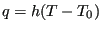(613)

where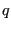is the a flux normal to the surface,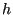is the film coefficient,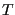is the body temperature and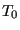is the environment fluid temperature (also called sink temperature). Generally, the sink temperature is known. If it is not, it is an unknown in the system. Physically, the convection along the surface can be forced or free. Forced convection means that the mass flow rate of the adjacent fluid (gas or liquid) is known and its temperature is the result of heat exchange between body and fluid. This case can be simulated by CalculiX by defining network elements and using the *BOUNDARY card for the first degree of freedom in the midside node of the element. Free convection, for which the mass flow rate is a n unknown too and a result of temperature differences, cannot be simulated.